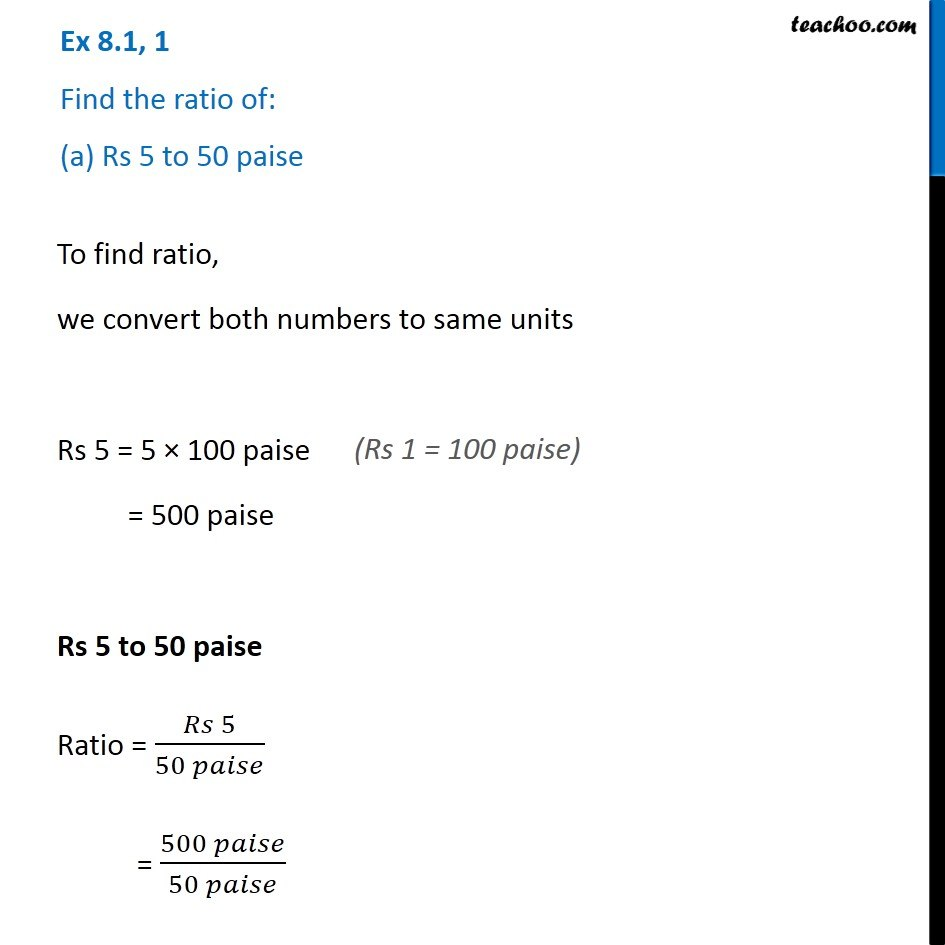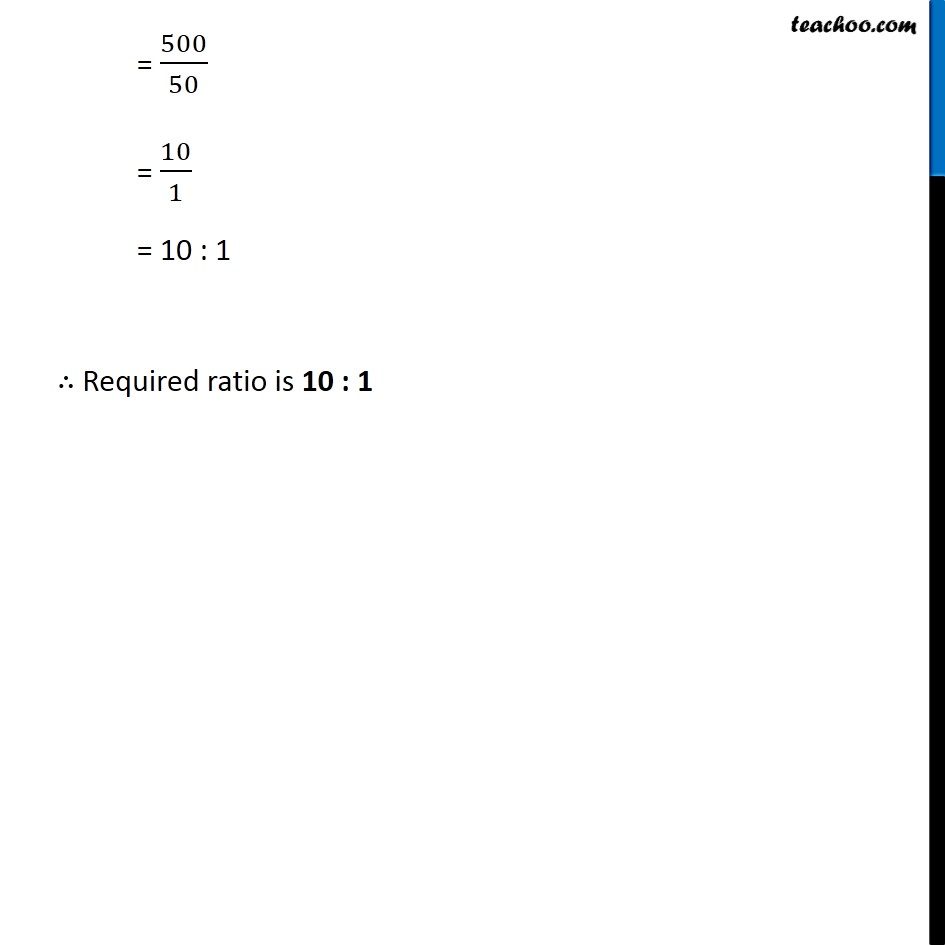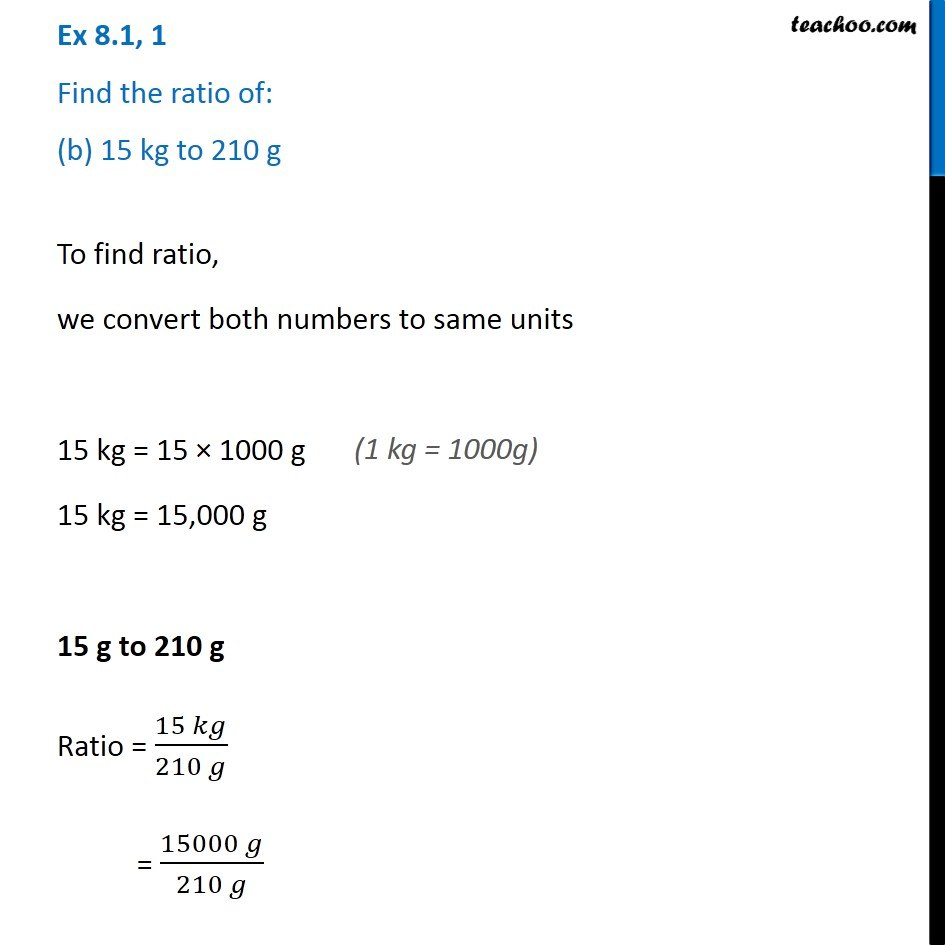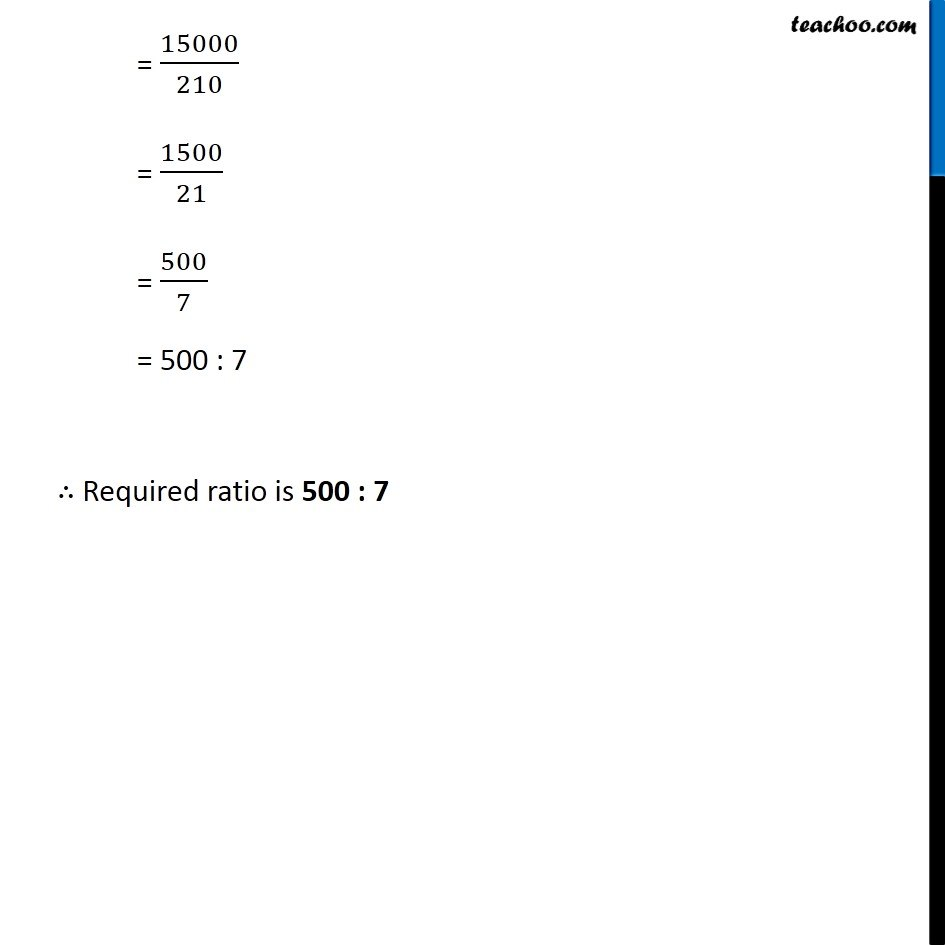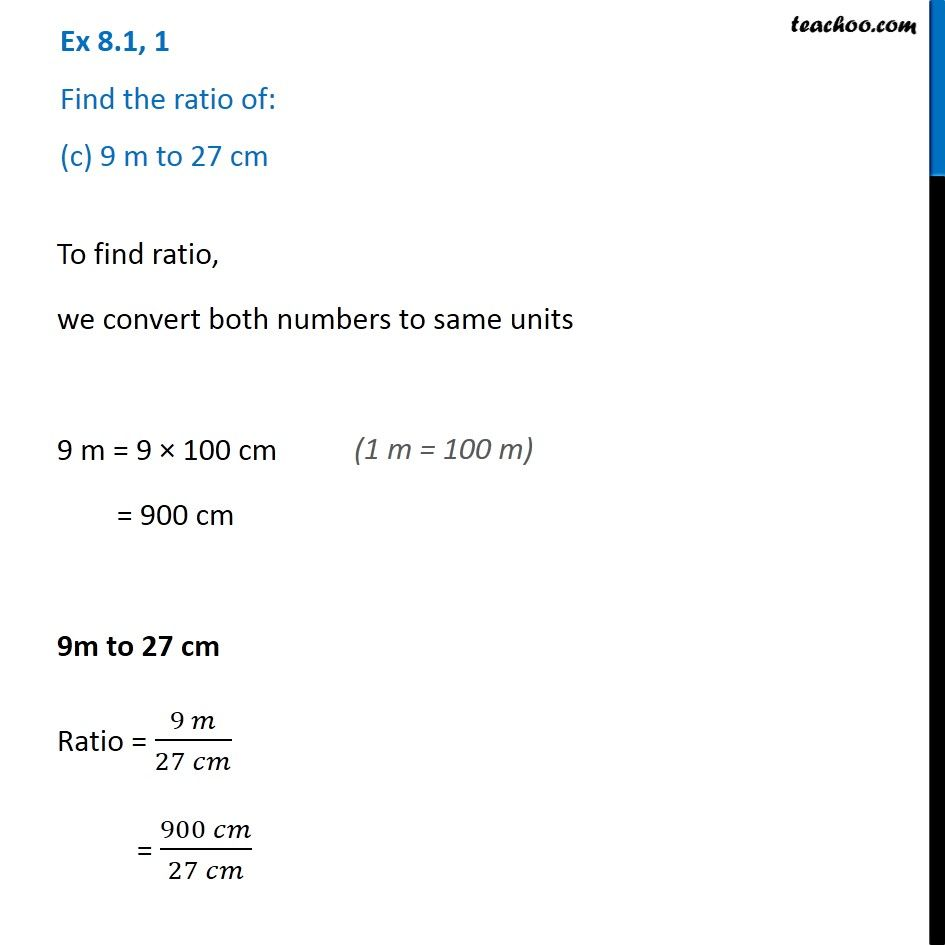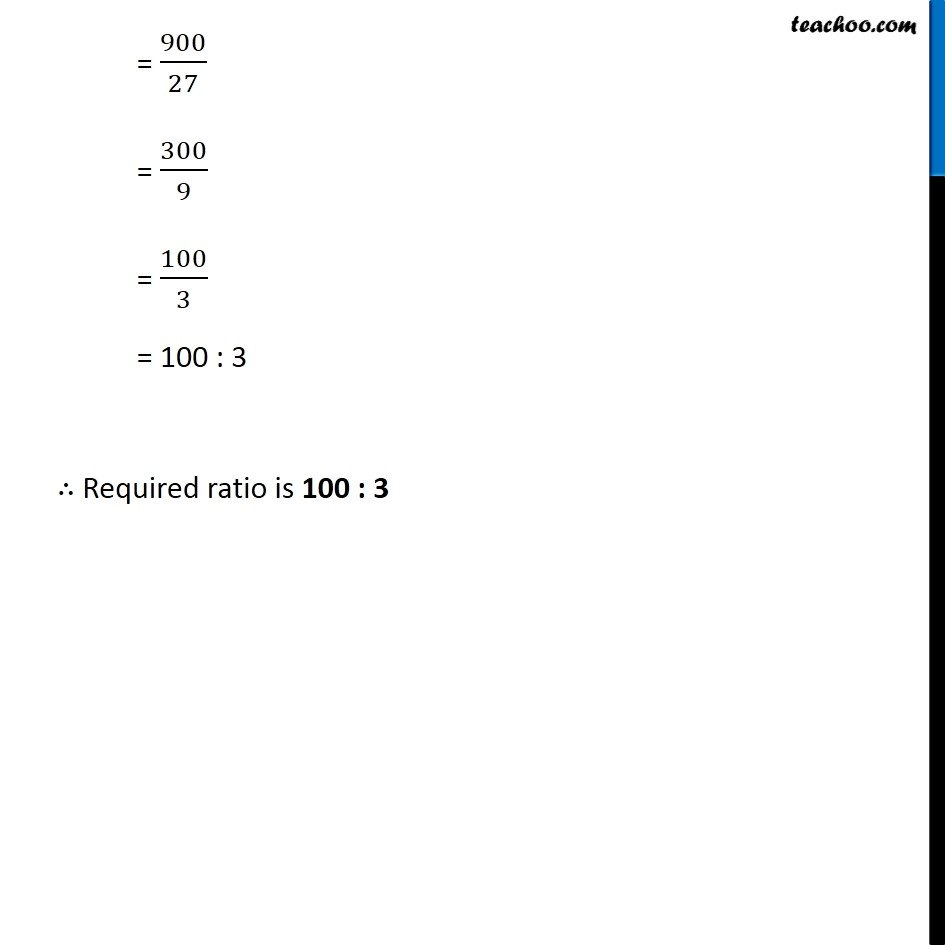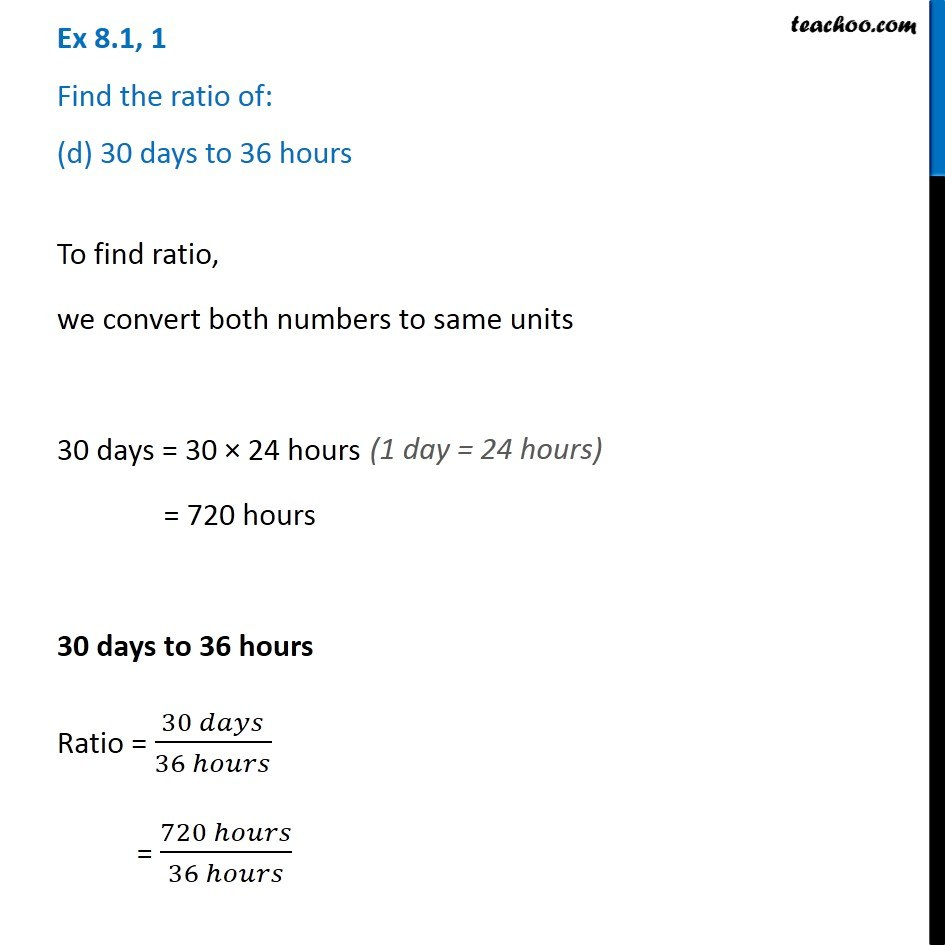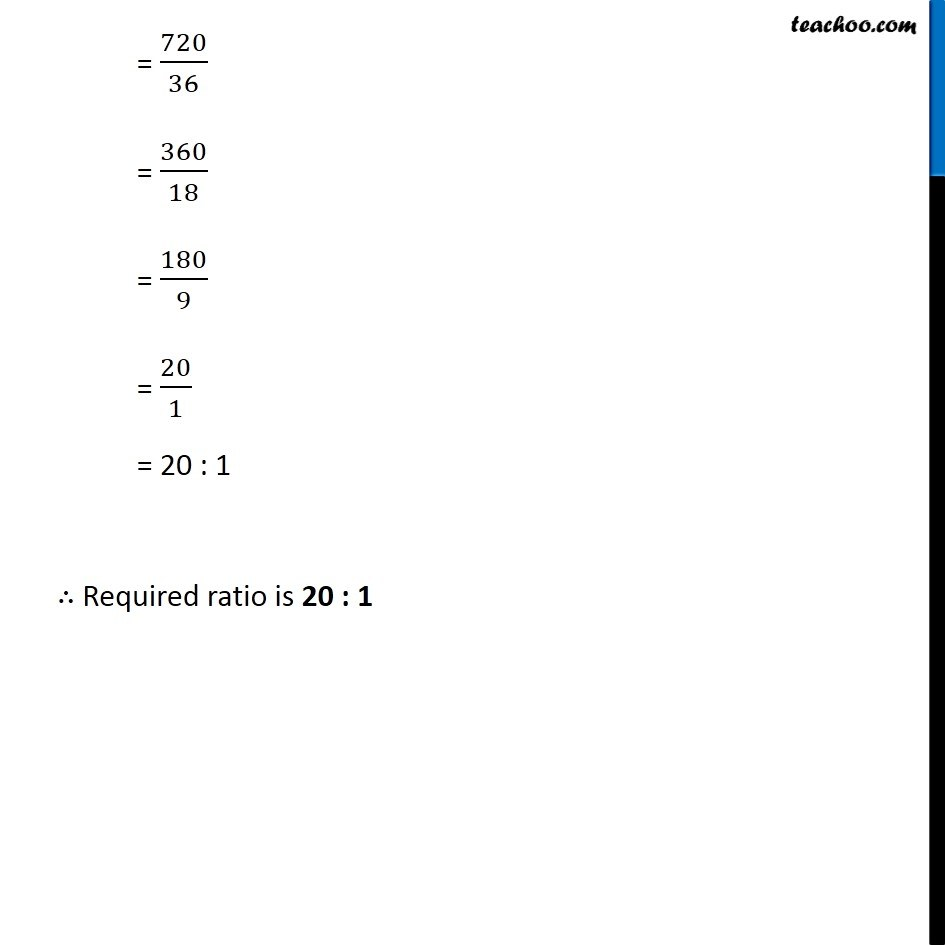Subscribe to our Youtube Channel - https://you.tube/teachoo

1. Chapter 8 Class 7 Comparing Quantities
2. Serial order wise
3. Ex 8.1

Transcript

Ex 8.1, 1 Find the ratio of: (a) Rs 5 to 50 paise To find ratio, we convert both numbers to same units Rs 5 = 5 × 100 paise = 500 paise Rs 5 to 50 paise Ratio = (𝑅𝑠 5)/(50 𝑝𝑎𝑖𝑠𝑒) = (500 𝑝𝑎𝑖𝑠𝑒)/(50 𝑝𝑎𝑖𝑠𝑒) = 500/50 = 10/1 = 10 : 1 ∴ Required ratio is 10 : 1 Ex 8.1, 1 Find the ratio of: (b) 15 kg to 210 g To find ratio, we convert both numbers to same units 15 kg = 15 × 1000 g 15 kg = 15,000 g 15 g to 210 g Ratio = (15 𝑘𝑔)/(210 𝑔) = (15000 𝑔)/(210 𝑔) = 15000/210 = 1500/21 = 500/7 = 500 : 7 ∴ Required ratio is 500 : 7 Ex 8.1, 1 Find the ratio of: (c) 9 m to 27 cm To find ratio, we convert both numbers to same units 9 m = 9 × 100 cm = 900 cm 9m to 27 cm Ratio = (9 𝑚)/(27 𝑐𝑚) = (900 𝑐𝑚)/(27 𝑐𝑚) = 900/27 = 300/9 = 100/3 = 100 : 3 ∴ Required ratio is 100 : 3 Ex 8.1, 1 Find the ratio of: (d) 30 days to 36 hours To find ratio, we convert both numbers to same units 30 days = 30 × 24 hours = 720 hours 30 days to 36 hours Ratio = (30 𝑑𝑎𝑦𝑠)/(36 ℎ𝑜𝑢𝑟𝑠) = (720 ℎ𝑜𝑢𝑟𝑠)/(36 ℎ𝑜𝑢𝑟𝑠) (1 day = 24 hours) = 720/36 = 360/18 = 180/9 = 20/1 = 20 : 1 ∴ Required ratio is 20 : 1

Ex 8.1